# Torque and Equilibrium

\$150.58

Code Number: P075910-torque

Brand: AELAB

#### Purpose:

1. Understanding the concept of force moment (torque). Prove that the torque depends on the force and lever arm.
2. How to define the lever arm.
3. Equilibrium in the torque . Prove the formula for torque.
- +

Experiment of torque and equilibrium includes:

1. Moment disc (or torque disc): Made of plastic, diameter 26cm. 33 holes to attach plastic pins. Holes are arranged in concentric circles. It has a mechanism of ball bearing for low friction and magnet to attach magnetic board.

2. Brass plumb

3. Ruller

4. Precision pulley

5. Weights: 50g x 20 (including 2 hangers)

6. Magnetic board

7. Stainless steel rod 60cm., dia. 10mm (2 pcs)

8. H-Shaped base.

- Understanding the concept of force moment (torque). Prove that the torque depends on the force and lever arm.

- How to define the lever arm.

- Equilibrium in the torque . Prove the formula for torque.

# THEORY AND PRACTICE

## Concept of Torque

Allow an object with mass m to move forward (linear motion). We need to apply a force F = m.a (a: acceleration m).

But for rotation (around an axis or a fixed point), we need a Torque M.

The formula:

M = F x d                     (1)

F: Applied Force on that rotating object

d: Lever arm.

M, F, d: are vector quantities. The direction of M follows the right hand rule as shown below.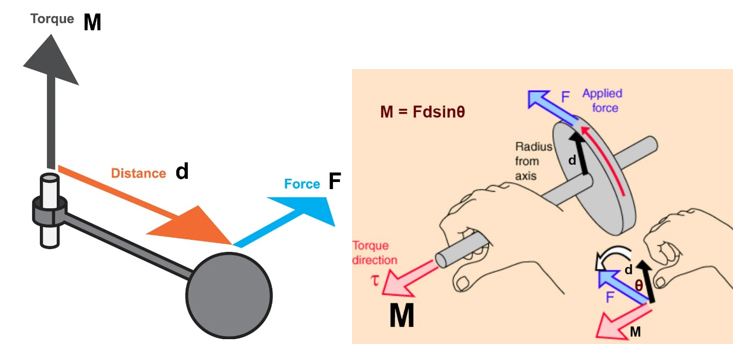If angle between the lever arm d and the F force is θ.

The magnitude of the force moment: M = F.d.sinθ

if θ = 90 degrees, then M = F.d

## Procedures

#### 1. To understand the concept of torque. Prove that the Torque depends on the force and lever arm.

See the clip below:

#### 2. How to define the lever arm.

Three ways:

+ Straight ruller;

+ Right triangle Ruller;

+ Adjust thread to tangent to the circle on torque disc (or moment disc).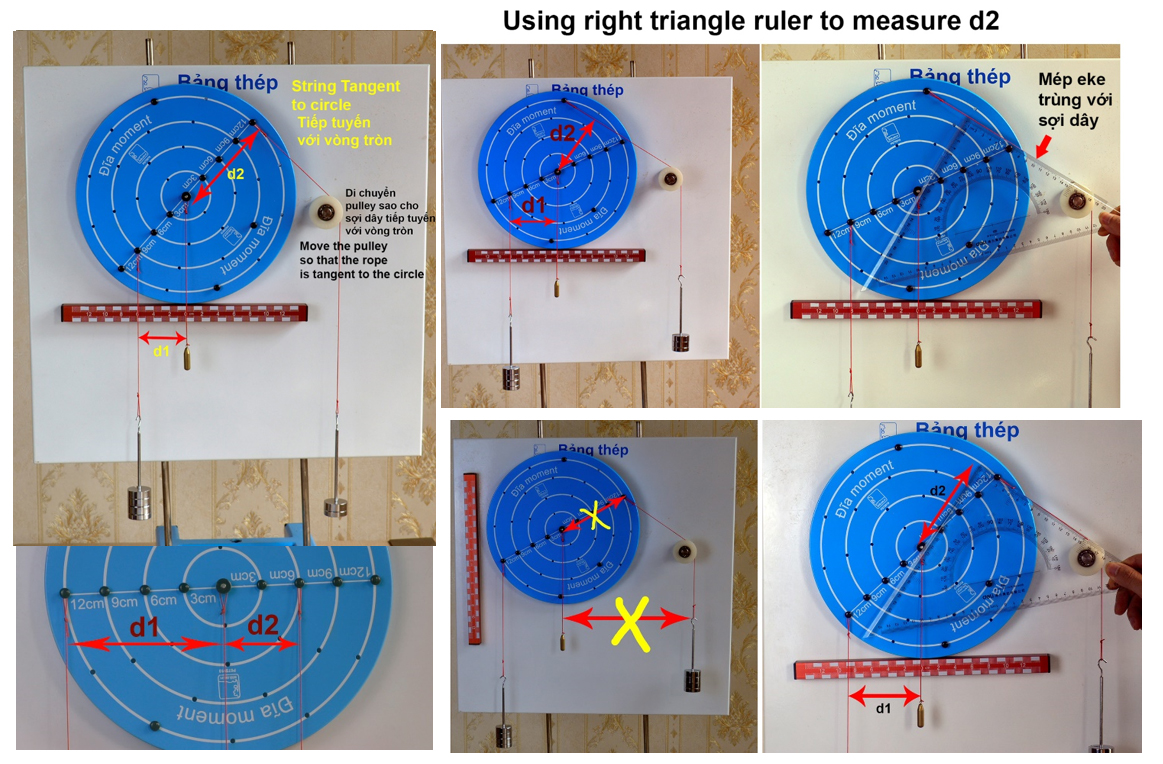#### 3. Equilibrium in torque. Prove the formula for torque.

An object in equilibrium of force will either stand still or move evenly.

With rotation, an object in equilibrium of torque do not rotate. This means that the torque acting to rotate in the clockwise direction will be equal to the torque that acts to rotate in the counter-clockwise direction.

These images in equilibrium of torque is below: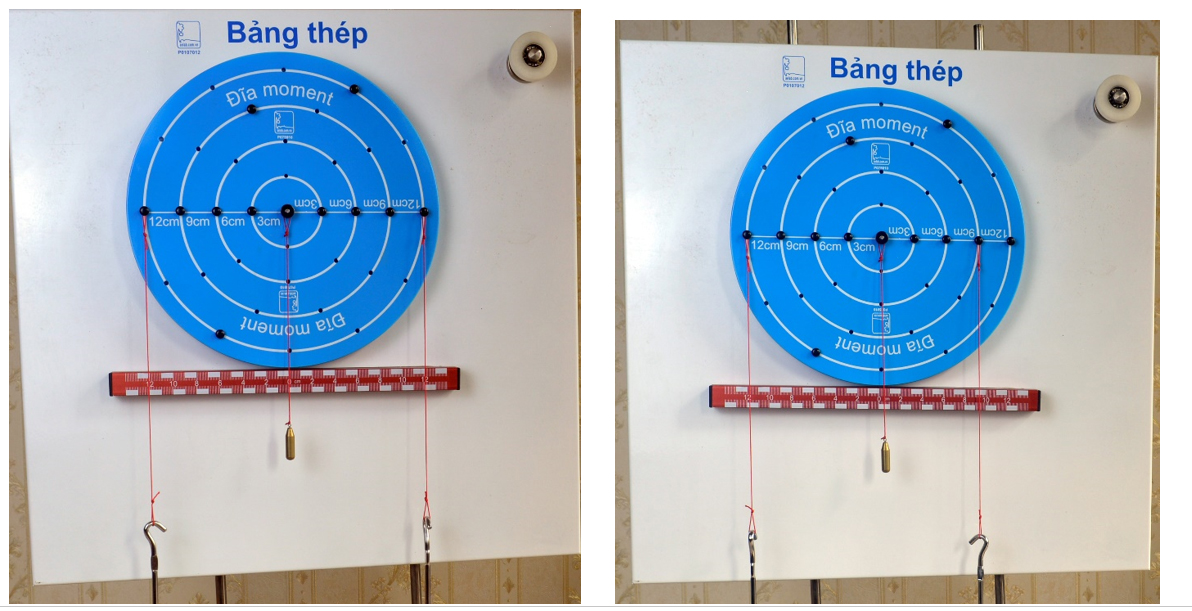See clip to know equilibrium and unequilibrium:

The specific analysis:

The force that causes the disc to rotate clockwise is: P2 and P3

The force that causes the disc to rotate counter-clockwise is P1

In Equilibrium: M1 = M2 + M3

P1.d1 = P2.d2 + P3.d3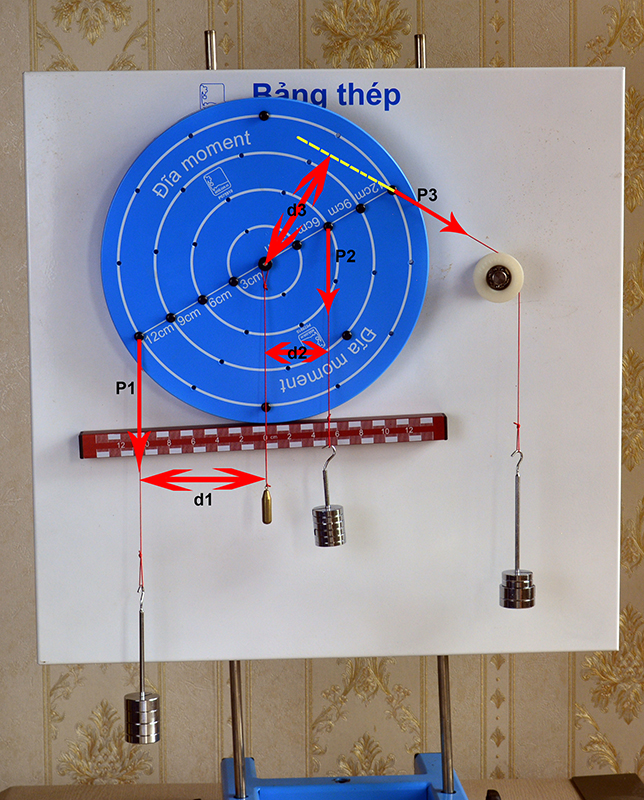In practice:

• With case: forces applied is parallel like picture below: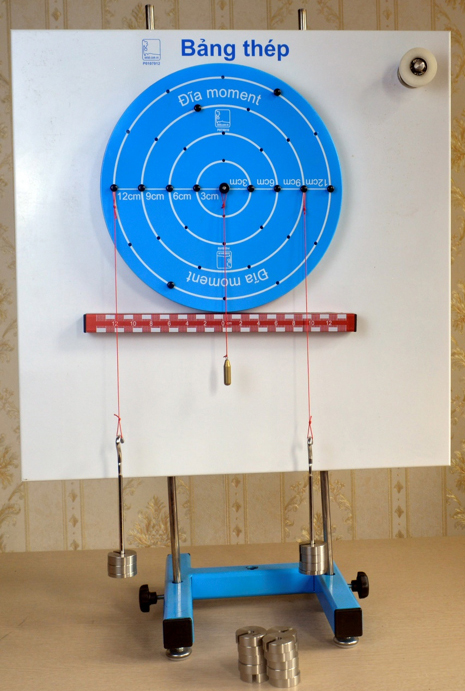Experiment of torque and equilibrium

We get data of experiment below: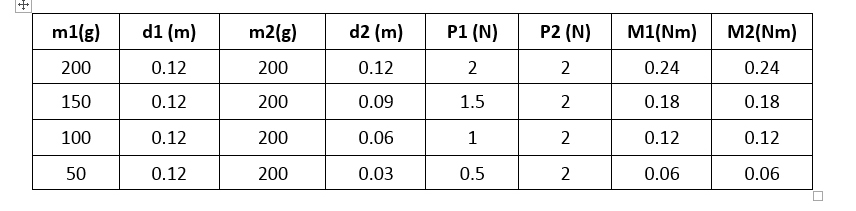Conclusion: In equilibrium: M1  = M2

• With case: forces applied is not parallel like picture below: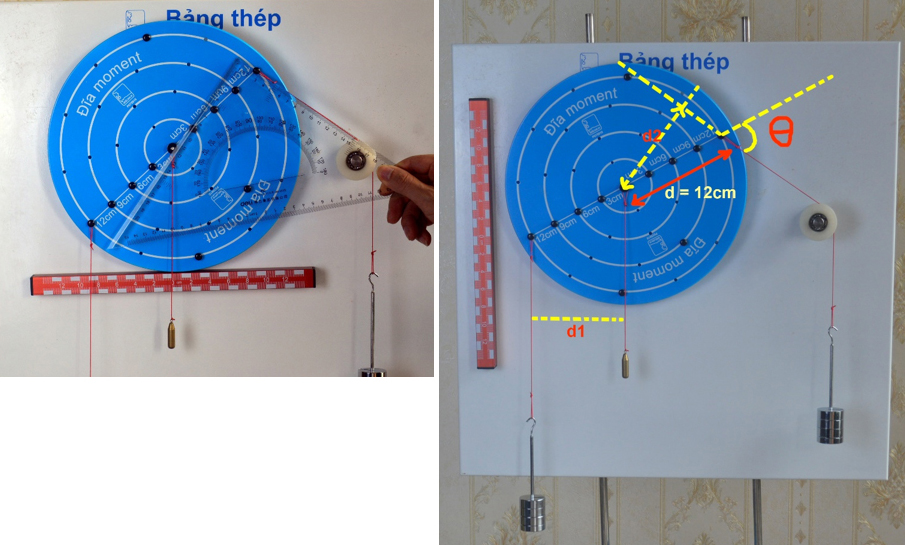Force F2 applies on disc at edge point with distance d = 12cm from center.

So we have formula: M2 = F2.d2 = F2.d.sin(θ).

And we have data of practice below: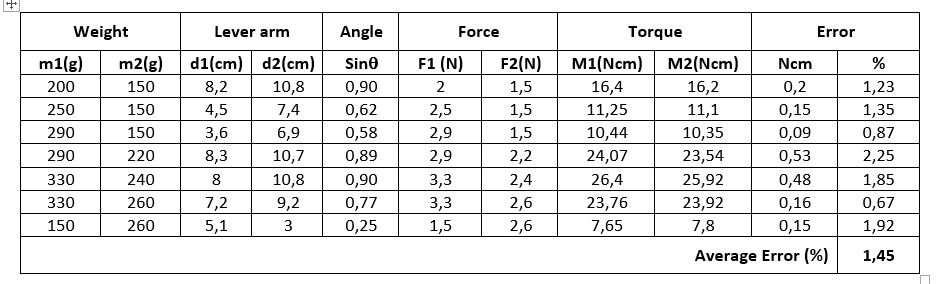In Equilibrium: M2 ~ M1

Average Error 1.45%.

The confirmation: in equilibrium, the total torque is zero. The torque formula is correct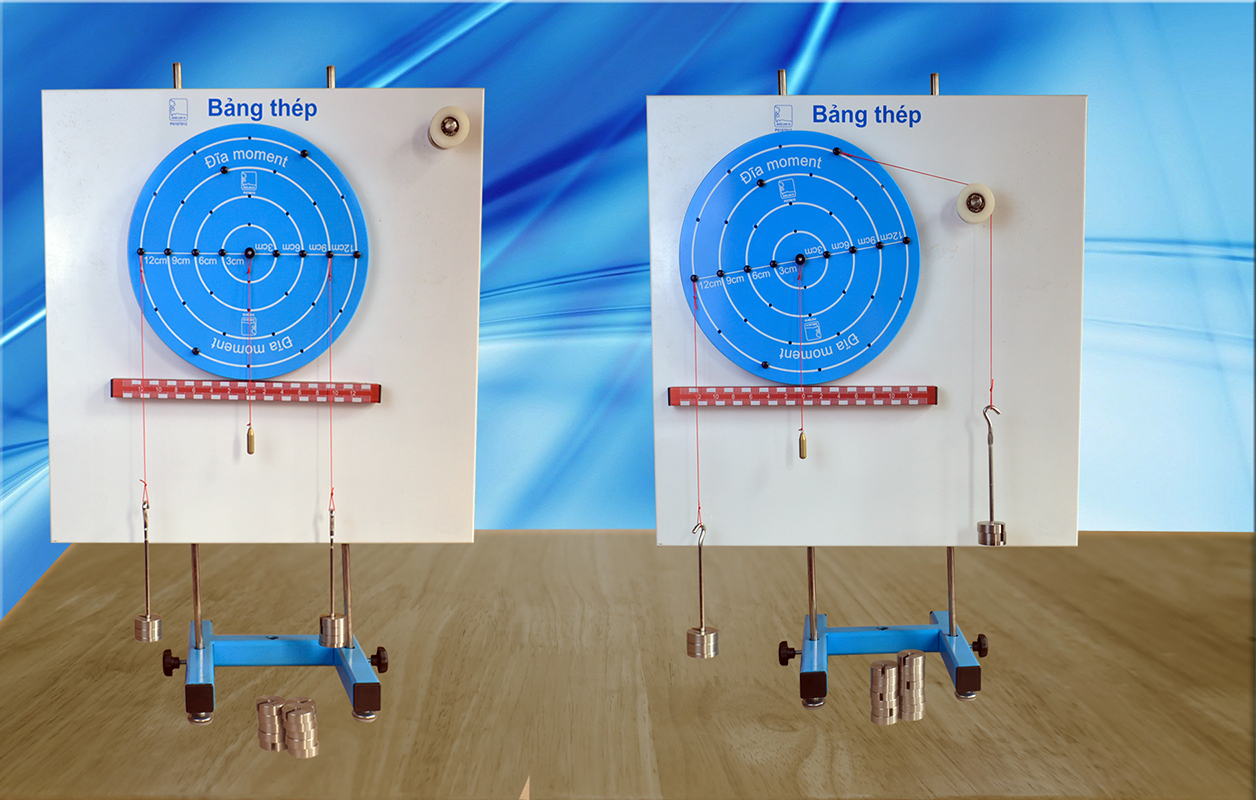Experiment of torque and equilibrium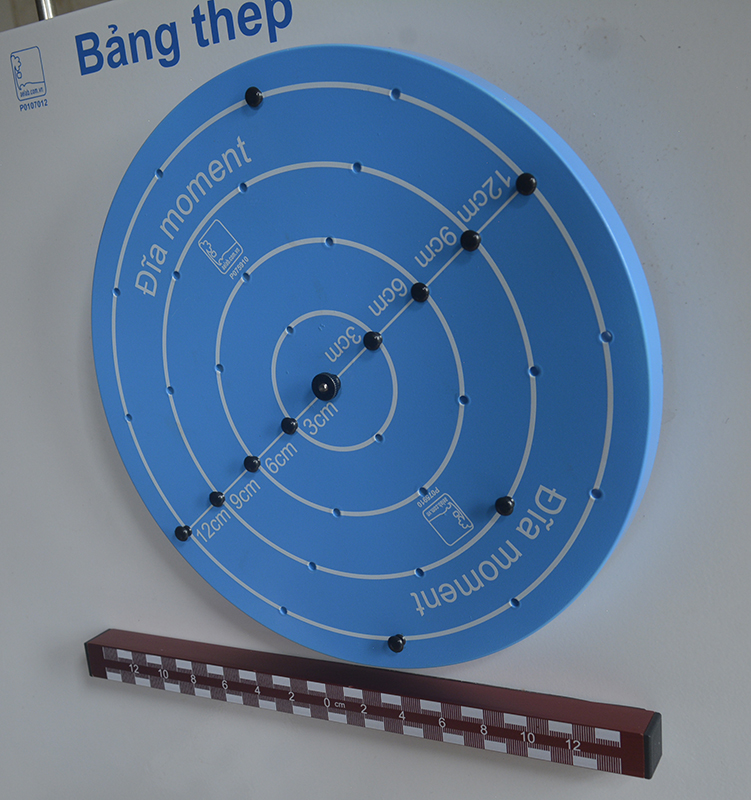Torque and Equilibrium experiment: Torque disc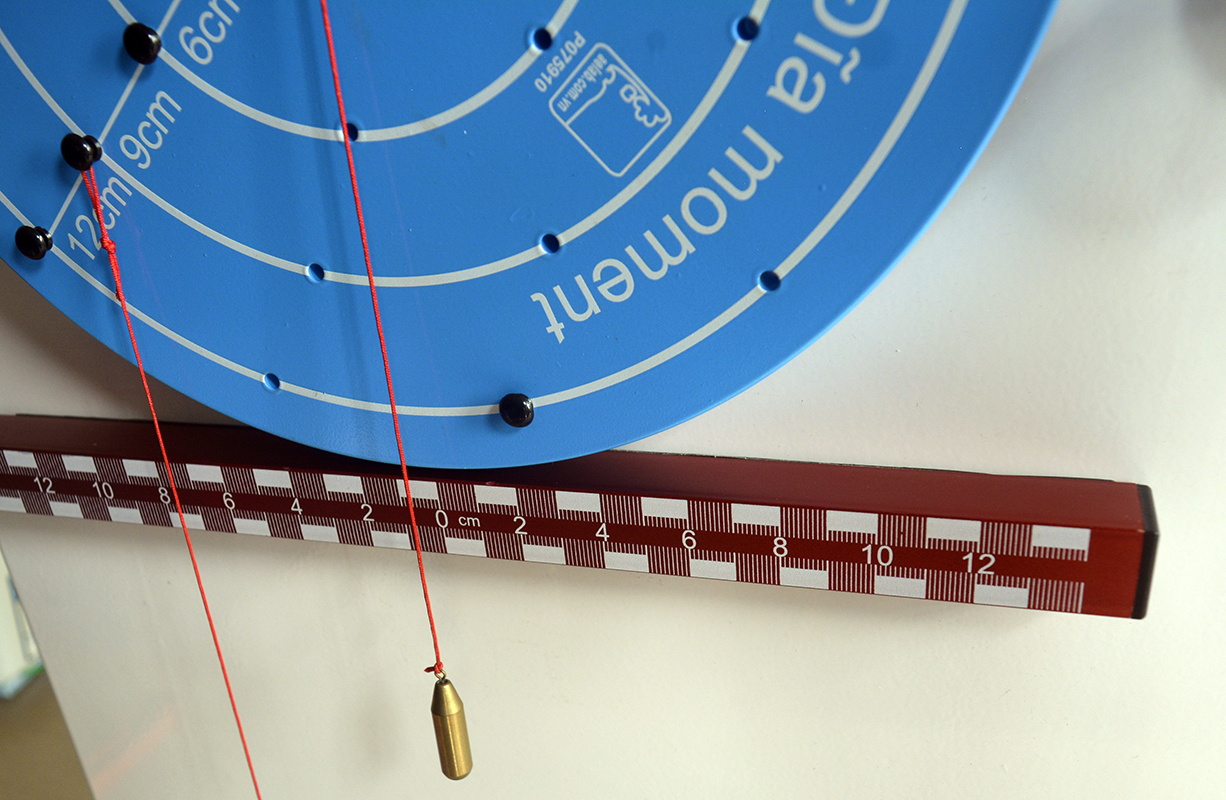Experiment of torque and equilibrium: Torque disc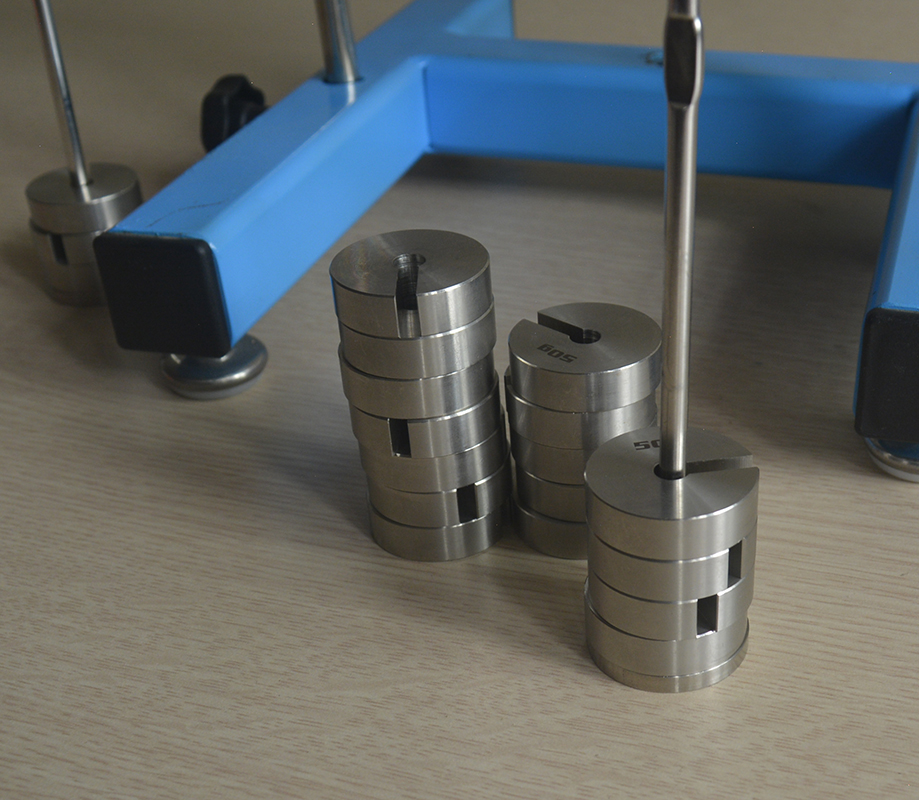Torque and Equilibrium experiment: weights

Test the friction of moment disc